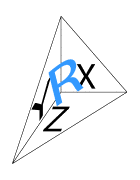By the end of high school, most students should be familiar with the “three-dimensional” Pythagorean theorem (aka, “the distance formula”) that relates the length of the diagonal of a shoebox to the lengths of its edges. (Students should also know that this generalization extends to relate diagonals and edges in “any-dimensional” shoeboxes; always, the square of the diagonal equals the sum of the squares of the edges at a corner.)

However, view students seem to be aware of this result:

Given a “right-corner tetrahedron”, the square of the area of the face opposite the right corner is equal to the sum of the squares of the areas of the other three faces.$$X^2+Y^2+Z^2=R^2$$

This result extends to “any-dimensional” space as simply as the distance formula: the square of the hypotenuse is equal to the sum of the squares of the legs. However, unlike the distance formula —which only ever relates one-dimensional lengths— the values being squared here are increasingly “dimensionally-enhanced” aspects of a figure: lengths in 2-d, areas in 3-d, volumes in 4-d, hyper-volumes in 5-d, and so forth.

I was thrilled as a high school junior when I discovered this result, and I was devastated as a college freshman when I discovered that others had beaten me to it by at least a century. Nevertheless, in the years —decades!— since, I’ve worked off and on to find related, completely new, results in the field I call “(Tetra)Hedronometry”, the dimensionally-enhanced trigonometry of tetrahedra. I’ve had a bit of success —in particular, in the realm of non-Euclidean geometry, where (so far as I can tell) absolutely nothing was previously known— and I believe my focus on tetrahedral “pseudo-faces” is a significant contribution to tetrahedral lore. We’ll see.

Posted 16 July, 2012 by in Hedronometry